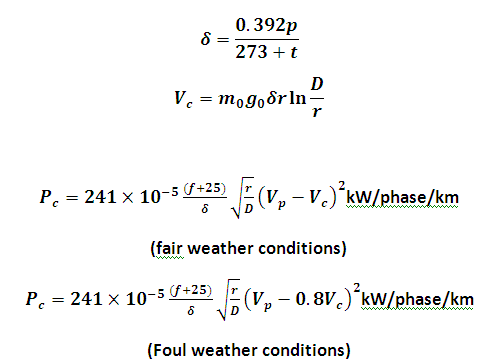# Corona Loss using Peek's Empirical relation Calculator

This Calctown Calculator calculates the corona loss using Peek's empirical realtion in a transmission system.

m
m
mm Hg
deg C
unit
Hz
kV

#### Result

kV
kw/phase/kmwhere

D = distance between conductors

δ = air density correction factor

p = pressure in Hg

t = temperature in 0C

Vc = corona inception or critical disruptive voltage

Vp = rms phase to neutral operating voltage

m0 = surface irregularity or stranding factor

g0 = breakdown strength of air = 21.2 kV/cm

f = frequency